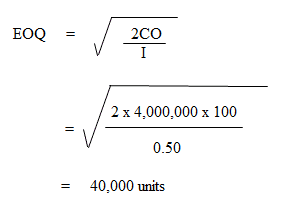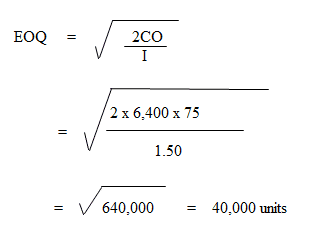## Problem 1: Calculation of Raw Materials to Produce Finished Products/Goods

A factory uses a specific raw material. There are also three processes A, B, and C. The data relating to inputs, outputs, and rejections for the month of April are given below.

 Inputs (in pieces) (including opening W.I.P) Rejections (in pieces) Output (in pieces) A 18,000 6,000 12,000 B 19,800 1,800 18,000 C 20,400 3,400 17,000

### Required

Calculate the cost of raw materials needed to produce one piece of the finished product when:

1. The weight of the finished product is 10 gram
2. The price of the raw material is \$1 per kg

### Solution

1. Process

 No. of Pieces Rejected Pieces Input Output No. % of output A 18,000 12,000 6,000 50% B 19,800 18,000 1,800 10% C 20,400 17,000 3,400 20%

If 1,000 pieces is the required output from Process C, the input should be 1,000 plus 20% (i.e., 1,200 pieces). This input of 1,200 units for Process C should be the output of process B.

The percentage of rejection, which is 10% in Process B, means that the input in Process B should be 1,200 pieces plus 10% (i.e., 1,320 pieces). Similarly, 1,320 pieces should be outputted by Process A.

With a percentage of rejection of 50%, the input of Process A should be 1,320 plus 50% (i.e., 1,980 pieces). This information can be tabulated as follows:

 Process Input Rejection % Rejection of Output Output A 1,980 660 50% 1,320 B 1,320 120 10% 1,200 C 1,200 200 20% 1,000

2. Given

The weight of the finished product is 10 grams per piece.

Assuming that there is no other loss of material, the total material required for 1,980 pieces of input for Process A is the following:

1,980 pcs. x 10 gms. = 19,800 gms.
Rate of Material = \$1 per kg
Cost of raw material = (19,800 x 1) / 1,000 = \$19.80
Cost of raw material per pc.= 19.80 / 1,000 = \$0.0198

## Problem 2: Calculation of Maximum, Minimum, and Reorder Stock Levels

(1) Discuss the consideration that influences the setting of maximum, minimum and reorder stock levels. Illustrate their computation by using the following information for a component ‘ZYP’.

 Normal Usage 50 per week Minimum Usage 25 per week Maximum Usage 75 per week Re-order Quantity 300 units Re-order Period 4 to 6 weeks

### Solution

Re-order level = Max. consumption per day / per week etc. x Max. re-order period
= 75 units x 6 weeks = 450 Units

Maximum Level = Re-order Level + Re-order Quantity – (Minimum consumption per day/per week etc. x Minimum time required to obtain supplies)
= 450 units + 300 units – (25 units x 4 weeks)
= 750 units – 100 units = 650 units

Minimum Level = Re-Order level – (Normal consumption per day/per week etc. x Average re-order period)
= 450 units – (50 units x 5 weeks)
= 450 units – 250 units = 200 units

(2) Two components, A and B, are used as follows:

• Normal usage = 50 units per week each
• Minimum usage = 25 units per week each
• Maximum usage = 75 units per week each
• Reorder quantity A: 400 units
• Reorder quantity B: 600 units
• Reorder period A: 4 to 6 weeks
• Reorder period B: 2 to 4 weeks

#### Required

For each component, calculating the following:

1. Reorder Level
2. Minimum level
3. Maximum level
4. Average stock level

### Solution

(1) Reorder Level = Maximum consumption per day/per week etc. x Maximum Re-Order Period
Component A = 75 units X 6 weeks = 450 units
Component B = 75 units X 4 weeks = 300 units

(2) Minimum Level = Reorder Level – (Normal consumption per day/per week etc. x Average Re-order period)
Component A = 450 units – (50 units x 5 weeks) = 200 units
Component B = 300 units – (50 units x 3 weeks) = 150 units

(3) Maximum Level = Reorder level – Reorder Quantity – (Minimum consumption per day/per week, etc. x Minimum Time Required to get supplies)
Component A = 450 units + 400 units – (25 units x 4 weeks)
= 850 units – 100 units = 750 units
Component B = 300 units + 600 units – (25 units x 2 weeks)
= 900 units – 50 units = 850 units

(4) Average Stock Level = Minimum Level + 1/2 (Reorder Quantity)
Component A = 200 units + (1 / 2 x 400 units) = 400 units
Component B = 150 units + (1/2 x 600 units) = 450 units

## Problem 3: Calculation of Material Turnover Ratio

The figures shown below were taken from the records of John and Co. for the year ended 31 March 2019. The valuation of inventory is \$1 per kg.

 Material ‘X’ Material ‘Y’ \$ \$ Opening Stock 1,700 1,200 Purchases 51,000 32,000 Closing Stock 1,200 1,000

### Required

Calculate the following:

1. Material turnover ratio
2. Number of days the average inventory is held

### Solution

(1) Material consumed

 Material X (kgs) Material Y (kgs) Opening stock Add: Purchases 1,700 51,000 1,200 32,000 52,700 33,200 Less: Closing Stock 1,200 1,000 51,500 32,200

(2) Average inventory
Average inventory = (Opening Stock + Closing Stock) / 2
Material X = (1,700 + 1,200) / 2 = 1,450 kgs.
Material Y = (1,200 + 1,000) / 2 = 1,100 kgs.

(3) Material turnover ratio
= Material consumed during the period / Average Inventory
Material X = 51,500 / 1,450 = 35.5 times (approx.)
Material Y = 32,200 / 1,100 = 29.3 times (approx.)

(4) Number of days average Inventory is held
= Total No. of days in the period / Material Turnover
Material X = 365 / 35.5 = 10.3 days (approx.)
Material Y = 365 / 29.3 = 12.5 days (approx.)

## Problem 4: Calculation of Economic Order Quantity

Consider the following information:

• Annual consumption: 40,00,000 kgs
• Cost of placing one order: \$100
• Cost of carrying one kg of raw material for one year: \$0.50

#### Required

Calculate the economic order quantity (EOQ).

####The annual demand for a product is 6,400 units. The unit cost is \$6 and the inventory carrying cost is 25% per annum.

#### Required

If the cost to procure one unit is \$75, determine the following:

1. EOQ
2. Number of orders per year
3. Time between two consecutive orders

#### Solution

1. Calculation of EOQ2. No. of orders per year = Annual consumption / Size of one order
= 6,400 units / 800 units
= 8 orders

3. Time gap between two consecutive orders = 12 months / No. of orders
= 12 months / 8 orders
= 1.5 months

### What is material costing?

Material costing is a system that measures and records the cost of material consumed during a specified period. It involves recording and monitoring raw materials used to produce finished goods and services.

### What is the formula of material cost?

Material cost = material cost / quantity * 100

### What is the most common problem in material cost accounting?

The most common problem faced by material cost accountants is that they collect too much data and do not analyze it effectively.

### What is the main purpose of material costing?

Determine the actual costs associated with producing products/services, so as to help managers make better decisions about pricing and allocating resources for future operations.

### What are the different types of material costing?

The two important methods of material costing are the first-in first-out method (fifo) last-in first-out method (lifo).

True is a Certified Educator in Personal Finance (CEPF®), a member of the Society for Advancing Business Editing and Writing, contributes to his financial education site, Finance Strategists, and has spoken to various financial communities such as the CFA Institute, as well as university students like his Alma mater, Biola University, where he received a bachelor of science in business and data analytics.

To learn more about True, visit his personal website, view his author profile on Amazon, his interview on CBS, or check out his speaker profile on the CFA Institute website.